# ALU

• ALU implementation in VHDL.
• The purpose of this repository is to perform logical operations such as addition and subtraction in the arithmetic unit and OR, AND, XOR in the logic unit. For this, two different encodings were made using the VHDL language in the Vivado program. One is implemented using component and accordingly the RTL schematics are also different. The same results were observed in the simulation.
• Vivado 2018.02 version was used in this project.
• You can read this file rapor.pdf for detailed information about the project.

## ALU Function Table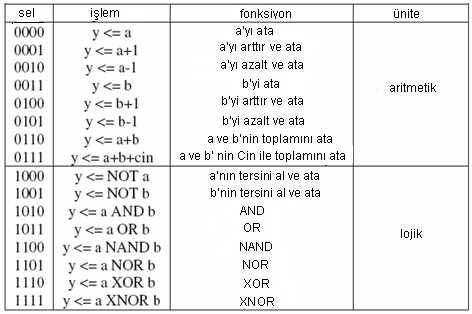## 1. ALU BLOCK DIAGRAM CREATED WITH THREE COMPONENT BLOCKS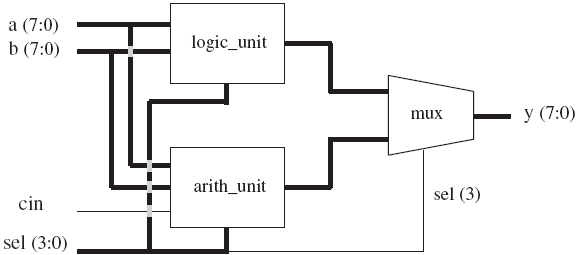### 1.1 RTL Schematic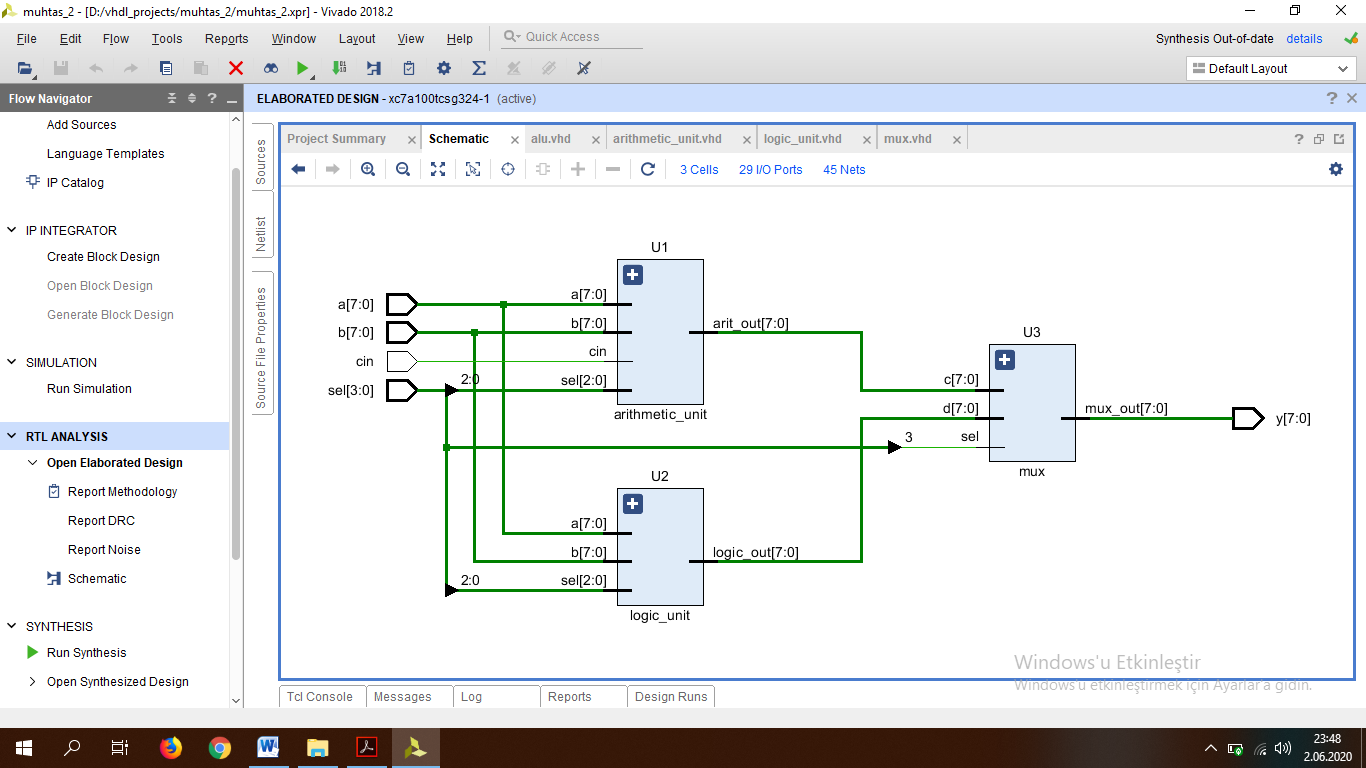### 1.2 Arithmetic Simulation Results

In the case of sel = “7”, that is, when sel = “0111”, y is equals a+b+cin.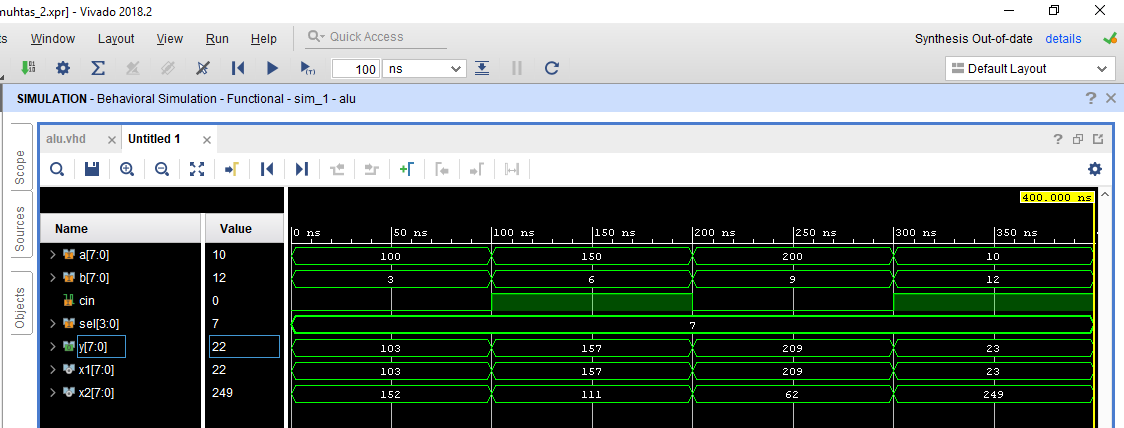In case sel= “1”, that is, when sel= “0001”, y is equals a+1. This was observed in the first 200ns. In the case of sel = “2”, that is, when sel = “0010”, y is equal to a-1. This situation was observed between 200ns-400ns.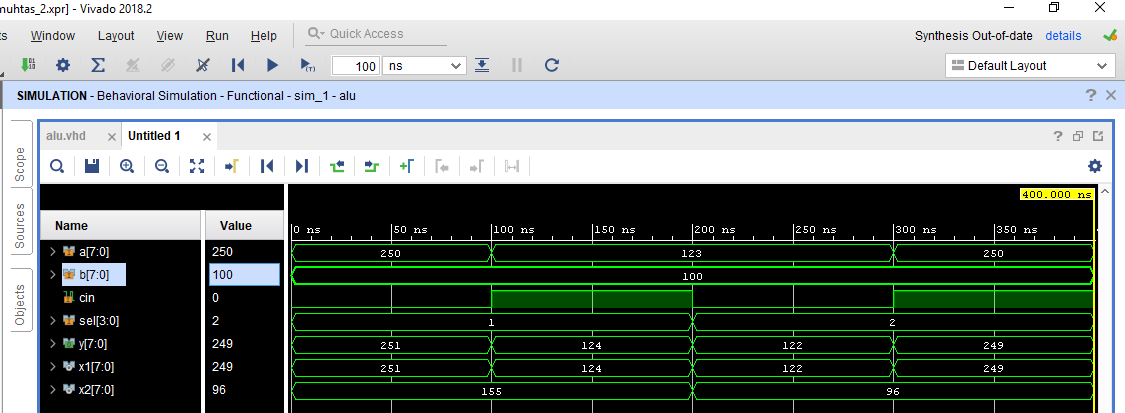### 1.3 Logic Simulation Results

In the case of sel= “10”, that is, when sel= “1010”, y is equal to “a AND b”.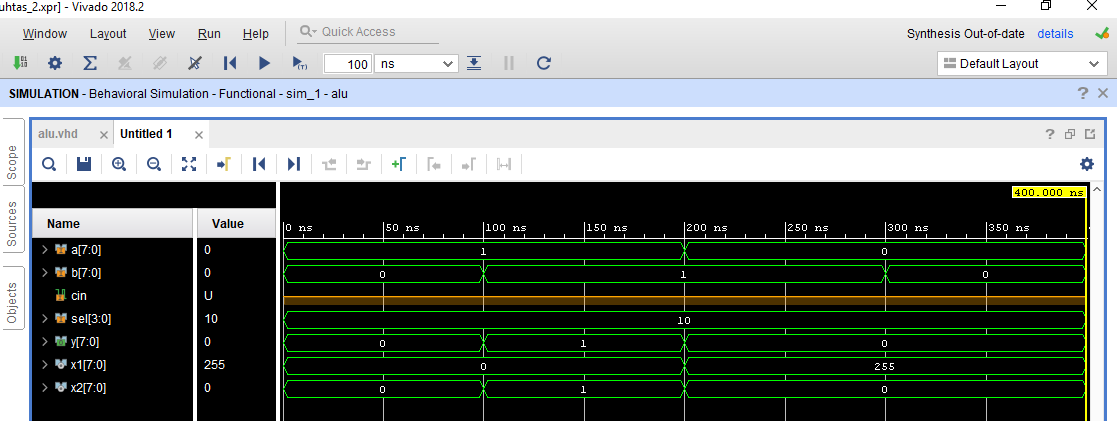In the case of sel = “11” that is when sel = “1011” yis equal to “a OR b”.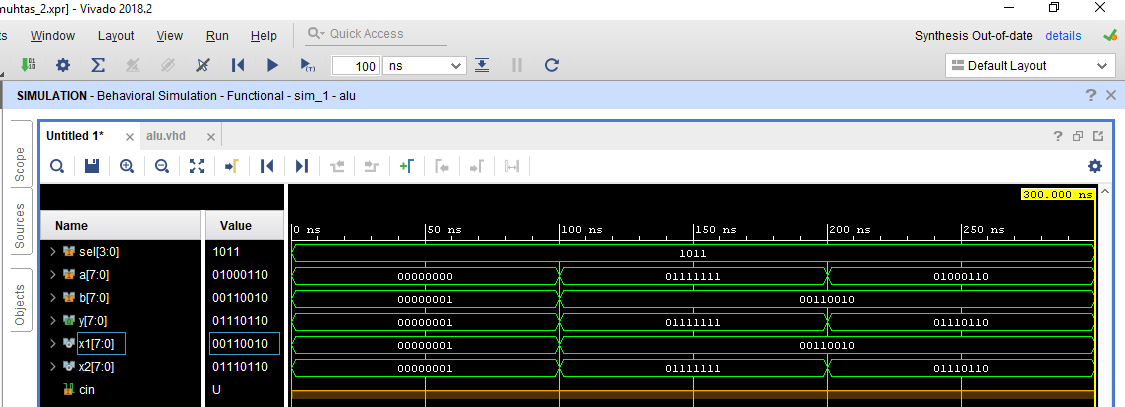## 2.COMPONENT-FREE ALU DESIGN

### 2.1 RTL Schematic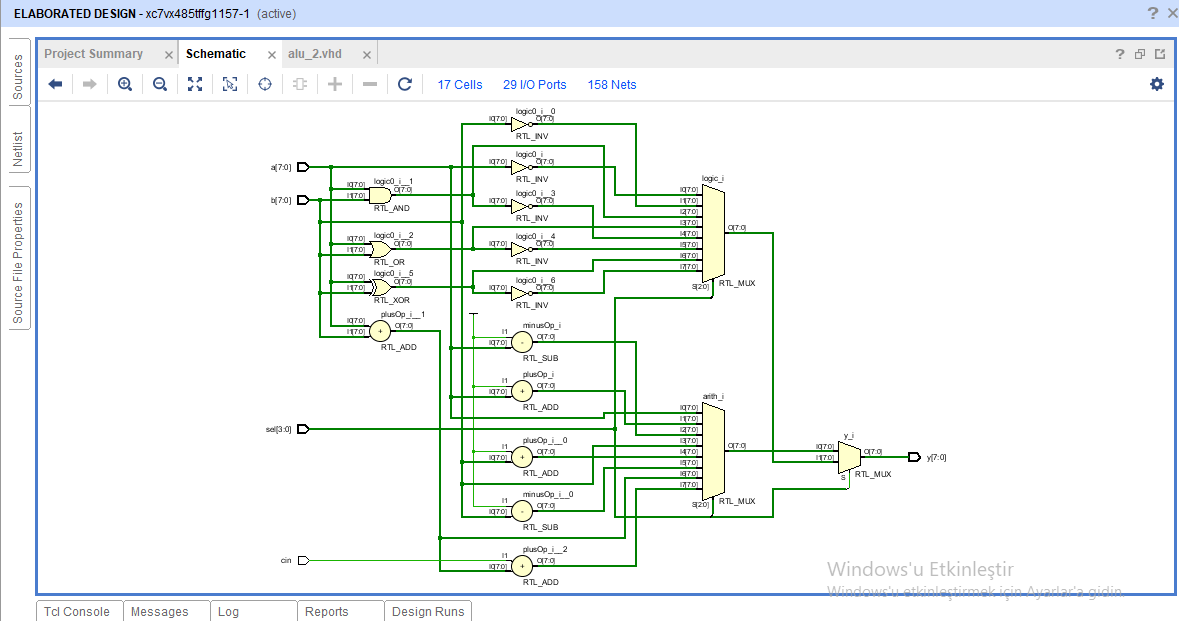View Github